Detecting and modeling non-proportional hazards in Cox regression

 Speakers:  Patrick Royston, Royal Postgraduate Medical School, and Peter Sasieni, Imperial Cancer Research Fund

Introduction

The Cox regression model attempts to quantify the relative hazard of an event, say death, at varying times after some time origin, such as diagnosis or initiation of treatment. The data consist of survival times, some of which are censored if the study ended before an event was observed for every patient, a censoring indicator variable which is 1 for events and 0 for censored observations, and usually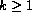covariates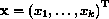. The hazard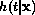of death at time t, given the values of the covariates, is represented as proportional to a baseline hazard function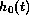: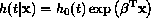where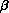is a vector of regression coefficients. In the estimation of the parameters,, the baseline hazard function does not need to be explicitly estimated. This flexibility is perhaps the strongest feature of the Cox model.

In most medical papers, the authors take the proportional hazards (PH) assumption for granted and make no attempt to check that it has not been violated in their study data. However, it is a strong assumption indeed. Suppose there was a hypothetical treatment which was quite effective in reducing the death rate from some condition during the first year after diagnosis, but whose effectiveness wore off rapidly after that, as the disease process reasserted itself. PH dictates that the treatment effect is constant on the relative hazard scale, so the estimated regression coefficient for treatment from the Cox model will actually represent some kind of time-averaged treatment effect. The important fact that the treatment effect is transient is undetectable within the standard Cox framework.

One approach to detecting non-PH is to extend the Cox model as follows: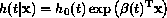Now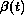is a function of follow-up time, thus allowing transient and other time-related covariate effects to be represented in an intuitive way. There are different ways of gaining an impression of the nature of. Two ways are described here. See Valsecchi et al. (1996) for further details and examples. The first way is to impose a `window' on the data and estimatelocally within the window according to a standard Cox model. The window is then shifted and the process is repeated. It is convenient to define each window as containing a fixed number of events (deaths), such as 10 × k. The local estimates of theand 95% confidence intervals, derived as ± 2 times the standard error, are then plotted against the central time point of each window to give an impression of the functional form. This approach is implemented in the ado-files stbtcalc and stbtplot. The second way is through quantities known as Schoenfeld residuals. The technical details are given by Gramsch and Therneau (1994) and are not required here. Again a local estimate ofis obtained, but this time it is essential to apply a smoother to get an impression of the functional form. We use running (Sasieni 1995; Sasieni & Royston 1998). The technique is implemented in the ado-files stgtcalc and stgtplot. Alternatively, a more restricted smoother such as fracpoly could be applied. Use of fracpoly (or other parametric models, such as regression splines) has added value in that it allows us to define, albeit heuristically, significance tests of non-PH.

Having identified that non-PH is present, the next step may be to try to modelparametrically. It requires the dataset to be expanded in a particular way, and a Cox regression with time-varying covariates must be estimated. We won't go into this here.

In the talk, we will give a computer demonstration of the use of the new ado-files in detecting non-proportional hazards in a clinical trial dataset. Some details are given in the next section.

Example: β2-microglobulin in myelomatosis

Myelomatosis is a cancer of the bone marrow which affects the immune system. One of the effects of the disease is the secretion by cancer cells of the protein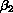-microglobulin (B2). A high serum concentration of B2 indicates advanced disease and is associated with a poor prognosis. The British Medical Research Council has carried out several controlled clinical trials of treatments for myelomatosis. We analyze data from the fourth and fifth trials (MacLennan et al. 1988). A total of 1087 patients were entered, of whom 571 had died by 913 days (about 2.5 years) after randomization, the latest time with complete follow up on all patients. We use Cox regression to analyze the relation between the B2 concentration at randomization and the log relative hazard of death.

Preliminary analysis reveals k = 4 covariates that are significant (P < 0.01) predictors of the hazard of death in a multivariable model. Although treatment effects were significant, the treatments in trials 4 and 5 were different and for simplicity we don't consider them here. The covariates are the patient's age (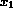), and the blood concentrations of-microglobulin (sb2,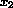), hemoglobin (hb,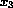) and calcium (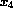). The following table shows the results of analysis using stcox.

. stset time cens

data set name:  myel45.dta
id:  --                    (meaning each record a unique subject)
entry time:  --                    (meaning all entered at time 0)
exit time:  time
failure/censor:  cens

. stcox c2 age sb2 hb calcium

failure time:  time
failure/censor:  cens

Iteration 0:  Log Likelihood =  -5353.41
[iteration log omitted]
Cox regression -- entry time 0

No. of subjects =         1050                    Log likelihood =  -5282.3789
No. of failures =          854                    chi2(4)        =      142.06
Time at risk    =  2659.268992                    Prob > chi2    =      0.0000

------------------------------------------------------------------------------
time |
cens | Haz. Ratio   Std. Err.       z     P>|z|       [95% Conf. Interval]
---------+--------------------------------------------------------------------
sb2 |   1.028496    .003653      7.911   0.000       1.021361     1.03568
hb |   .9932634   .0015708     -4.274   0.000       .9901894    .9963469
calcium |   1.363158   .1127263      3.746   0.000       1.159194     1.60301
age |   1.014327    .004299      3.356   0.001       1.005936    1.022788
------------------------------------------------------------------------------

All the chosen covariates have effects significant at the 0.1% level. Figure 1 shows the graphical output from the stbtplot command, created using the following commands:

. stbtcalc c2 sb2 hb calcium age
. stbtplot sb2 hb calcium age, xlabel(0,1,2,3,4,5,6,7) ylabel ci border

We have omitted results for c2 from the plot to improve legibility. Although there is some noise in each plot, the effect on the hazard is fairly stable over time. The effects of sb2, hb, age and calcium appear time-varying, the least so for calcium. The effect of sb2 seems to reach a peak after about 3–6 months and dwindle thereafter.

Figure 2 shows the graphical output from the stgtplot command, created using the following commands:

. stset time cens
. stcox c2 age sb2 hb calcium
. stgtcalc
. stgtplot sb2 hb calcium age, xlabel(0,1,2,3,4,5,6,7) ylabel ci border

Notice that you must call stcox before stgtcalc, because stgtcalc (unlike stbtcalc) is a post-estimation command. The plots provide an estimate ofup to nearly 7 years of follow up—in Figure 1,is estimated only up to t = 5 years. Although the smoothing has not removed all the noise, the impression again is that all the effects are time-varying. Qualitatively, the two sets of plots agree quite well, though the effect size generally seems larger with the stbtcalc approach. When in doubt we should probably trust the results from stbtcalc more than those from stgtcalc, since the stbtcalc estimation method is direct and should not produce much bias.

We can confirm the subjective impressions from Figure 2 parametrically by fitting first degree fractional polynomials to estimatefrom the Grambsch–Therneau (GT) residuals and applying a hypothesis test of no effect. The GT residuals are quietly created by stgtcalc as new variables whose names begin with the prefix GT. For example, the GT residuals for sb2 are stored in GTsb2. We would enter

. fracpoly regress GTsb2 time, degree(1) compare

The results show that there is a nonlinear effect of time on GTsb2, since the deviance difference between the first degree fractional polynomial model and the null model is 23.18. The deviance difference is significant at P < 0.001 when compared with chi-squared on 2 df. The first degree fractional polynomial, which has estimated power 0.5, fits significantly better than a straight line (P = 0.023). The same technique applied to age, hb and calcium gives P values of 0.037, 0.45 and 0.13, showing significant non-proportional effects of age but not of hemoglobin or calcium.

References

Grambsch, P. M. and T. Therneau. 1994.
Proportional hazards tests and diagnostics based on weighted residuals. Biometrika 81: 515–526.
MacLennan, I. C. M., K. Kelly, R. A. Crockson, E. H. Coopler, J. Cuzick, and C. E. Chapman. 1988.
Results of the MRC myelomatosis trials for patients entered since 1980. Hematological Oncology 6: 145–158.
Sasieni, P. 1995.
sed9: Symmetric nearest neighbor linear smoothers. Stata Technical Bulletin 24: 10–14.
Sasieni, P. and P. Royston. 1998.
sed9.1: Pointwise confidence intervals for running. Stata Technical Bulletin 41: 17–23.
Valsecchi, M. G., D. Silvestri, and P. D. Sasieni. 1996.
Evaluation of long term survival: use of diagnostics and robust estimators with Cox's proportional hazards models. Statistics in Medicine 15: 2763–2780.